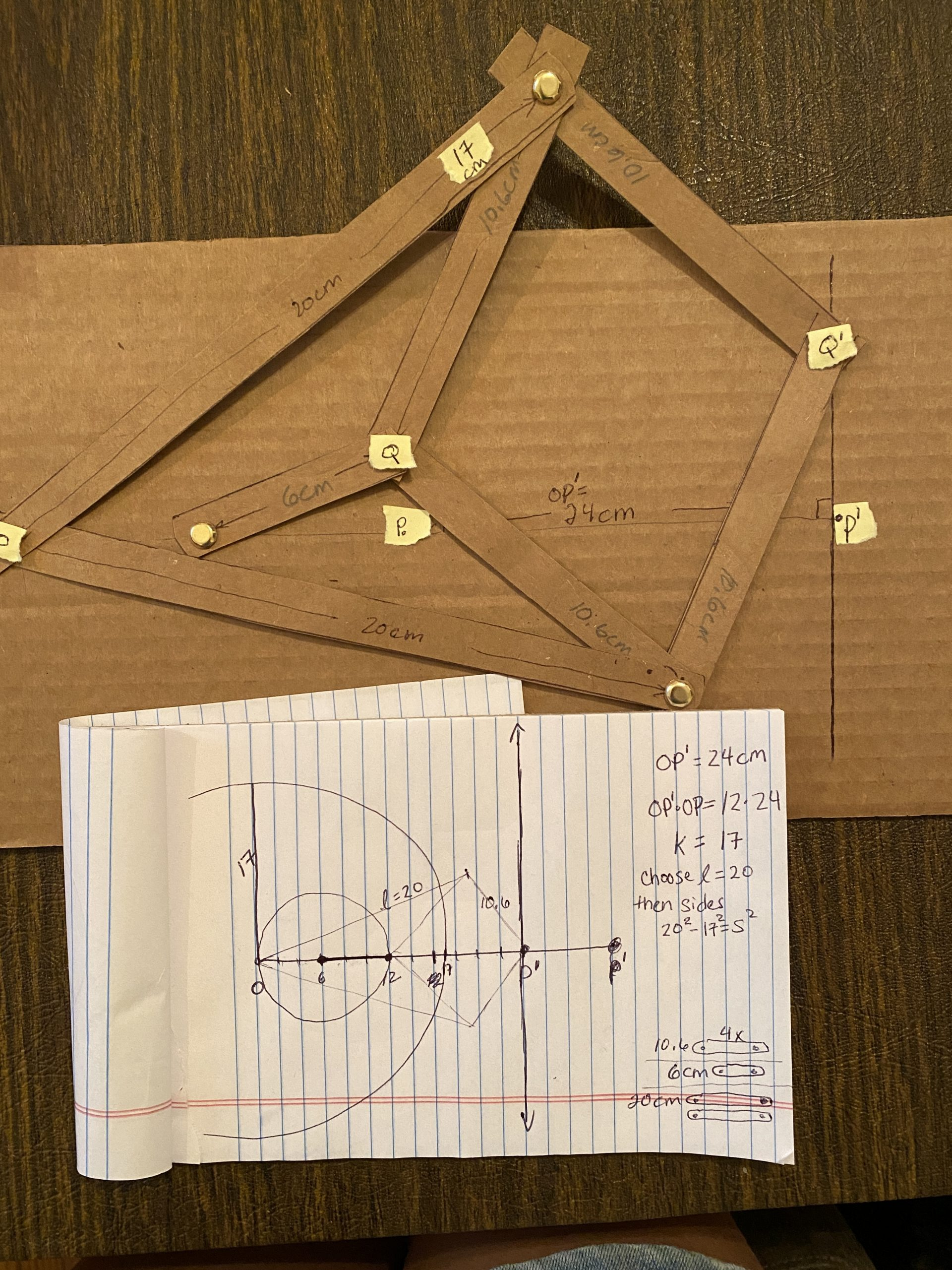# Finally Making a working Peaucellier Linkage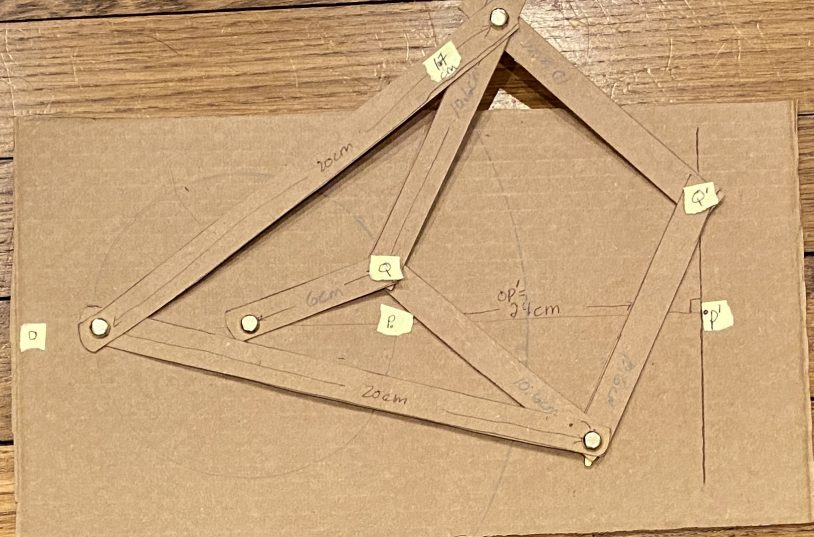Why does this project NEVER turn out?  It happens that we have been missing a critical fact.  The arm of the linkage is NOT the same length as the radius (k) of the larger reference circle used for the inversion even though we wanted it to be.  The workshop is littered with failed tries.  The arm length and the sides of the rhombus are related.  To make your own, you can copy our exact dimensions or do the calculations and make your own unique design.

Basic principal:  In the Geometry of Inversions, there’s a reference circle with radius k.  If there’s a small circle with a perimeter that intersects the center of the larger circle, we can show that the perimeter of the small circle will map via inversion onto a vertical line outside the larger circle.  A great explanation can also be found in Art of Problemsolving.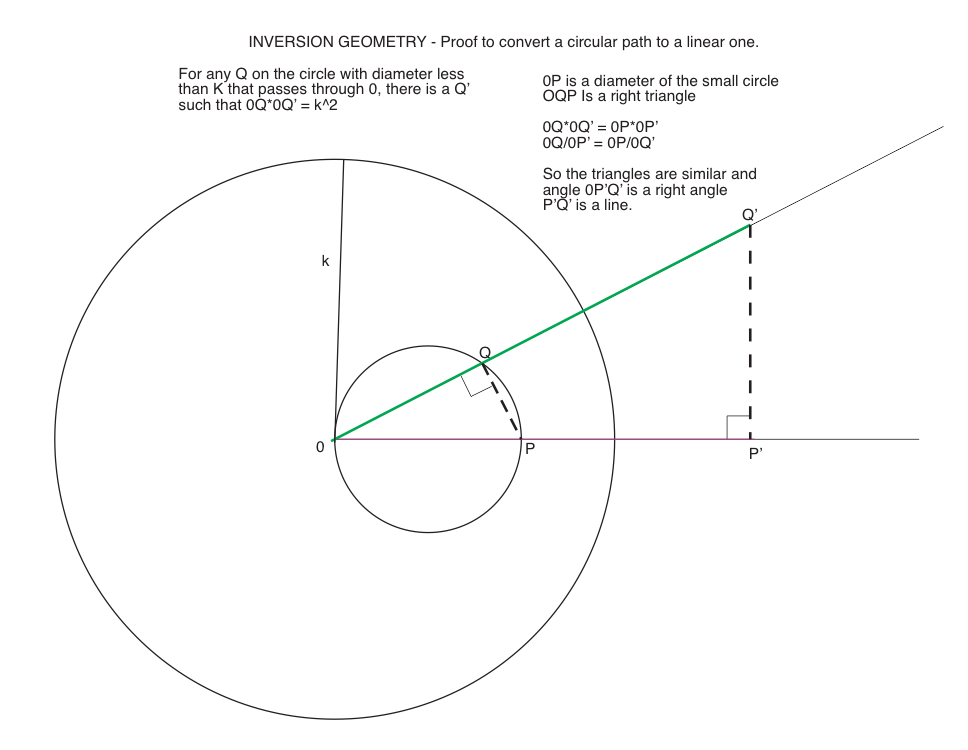It seems logical to locate the hinges of the arms of the inversion device on the perimeter of the larger circle, but this is not right.

A great explanation of this and other linkage machines can be found 30 minutes into this youtube video.

I put some notes based on that lecture in this Coreldraw sketch.  The arm (OA) is longer than the radius of the reference circle.  In fact, arm length ^2 = radius larger circle ^2 + side of rhombus ^2.  If you like, start at the left and wander through this proof that mostly depends on similar triangles, the Pythagorean Theorem and substitutions.  The two rigid arms keep the rhombus centered with the radius, but the rhombus can move to keep the end point on the vertical line.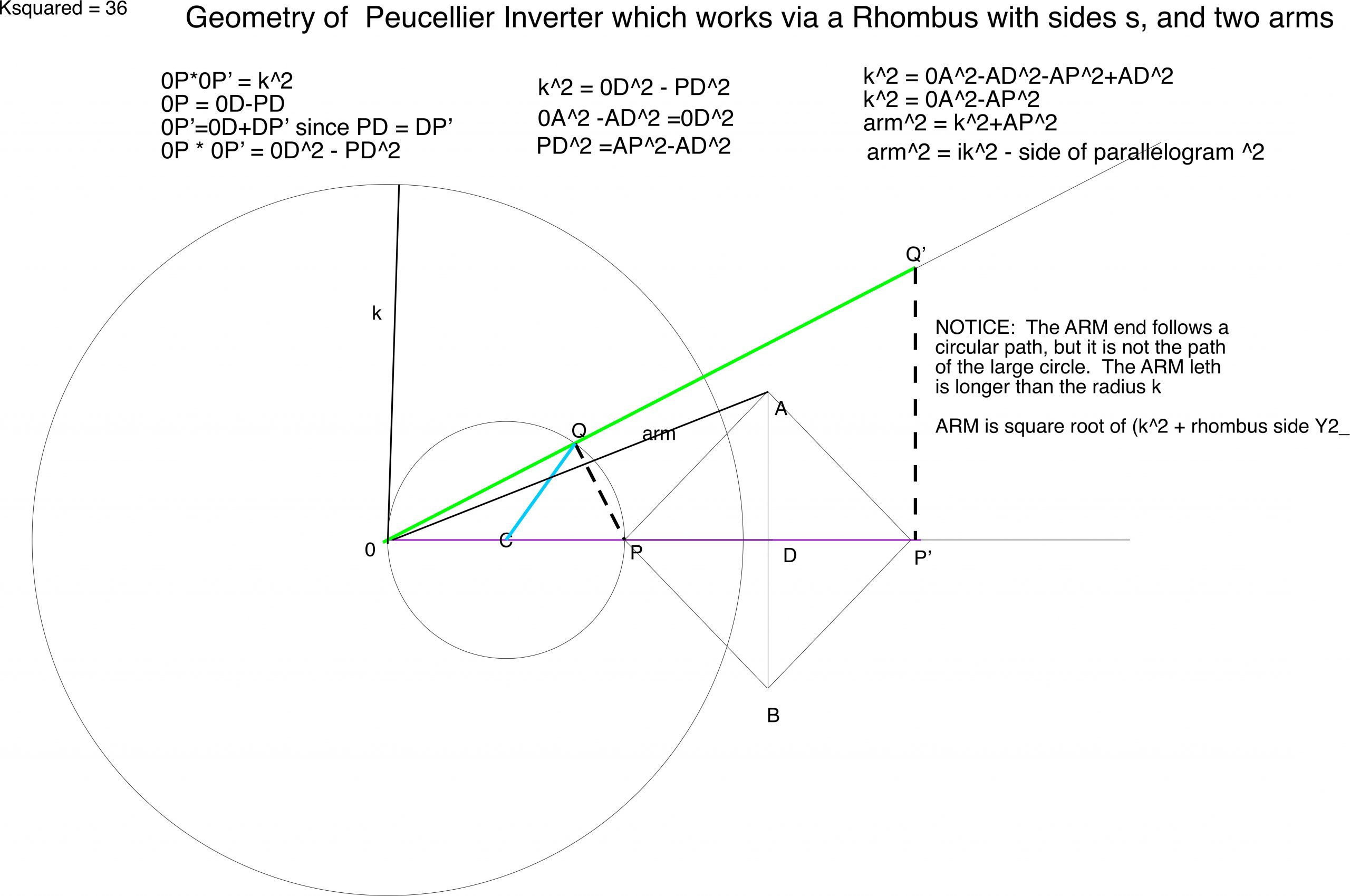So lets build one!  Choose your size.  I wanted mine to be 24 centimeters from the zero point on the left to the right side where we will have the vertical line (OP’) because that’s how big my cardboard is.  OP’ = 24.  Arbitrarily, but conveniently for space to draw and build links, I drew a reference circle with k (radius) = 17.  Note that I could pick the small circle first, then find the reference circle – either way works.  17^2 = 289 so 24 * OP must be = 289 and OP must be 12.  So now I have 0P*0P’= k^2 which is 12*24.

Next I also have choices for the the length of the arm, or I can choose the side length of the rhombus.  They are related, but many combinations work.  If I choose arm = 20cm, then the sides of the rhombus will be square root of (20^2-17^2) = 10.6

Next Make the linkages:  I need two holes in the base 24 cm apart at 0 and P’. And 4 links for the rhombus with holes 10.6cm apart.  I need two arms with holes 20 cm apart and one rotation arm that has holes 6cm apart.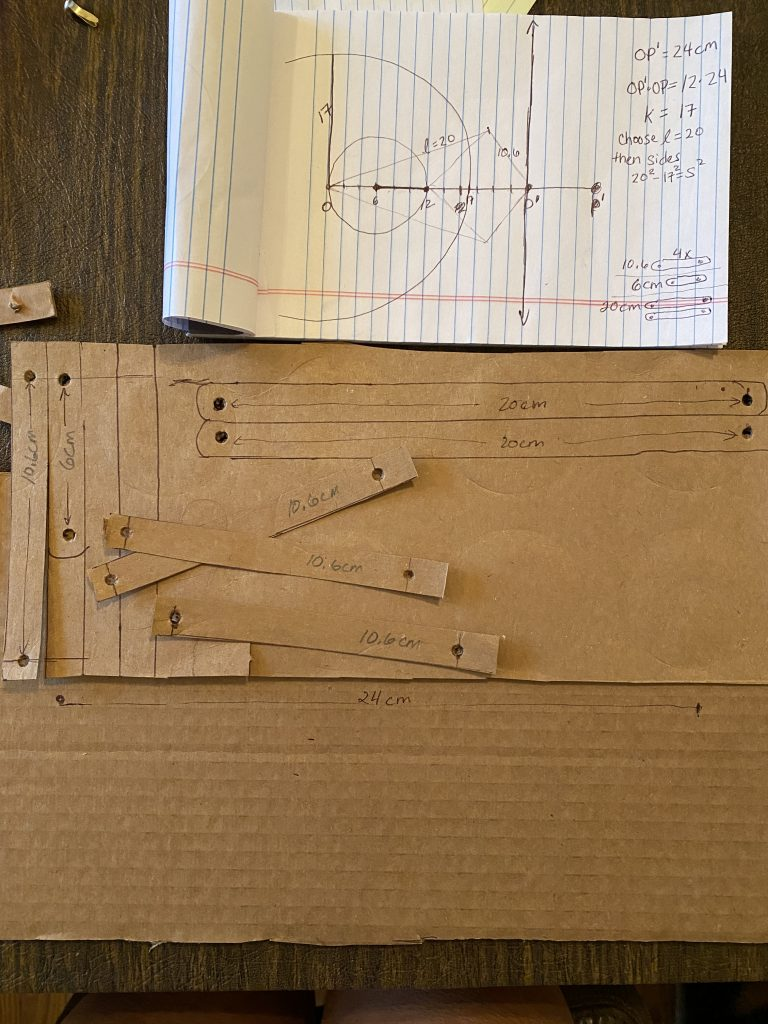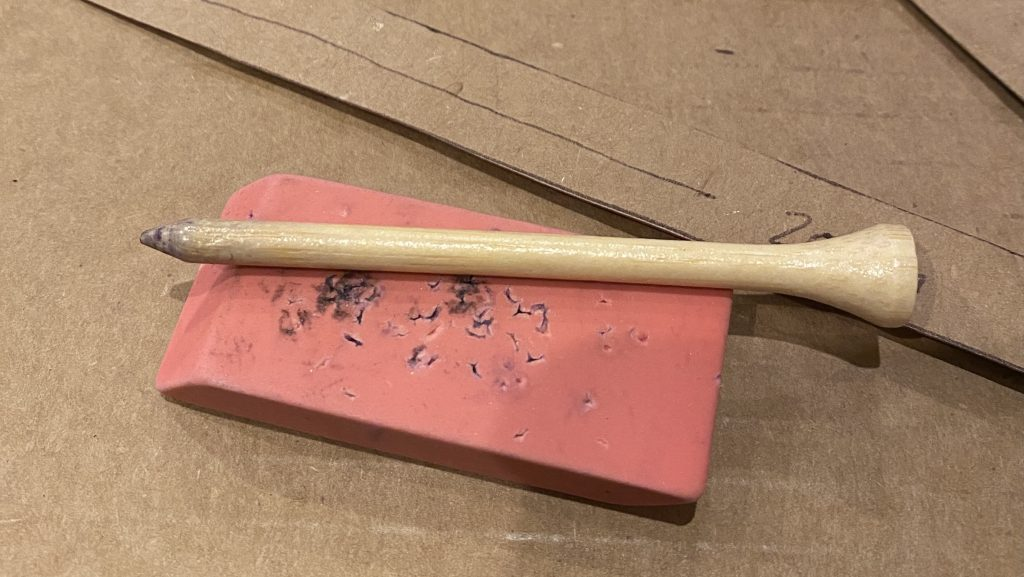Use an eraser as a base and golf tee to poke holes.  I used cardboard box for the base and cereal boxes for the linkages because my brads are short.

Put it all together!  It works beautifully and a multiple variations will work too.  Just remember, 17 cm is the radius of that larger reference circle, but a larger value, in this case 20 cm is the size needed for the arm.